# Algebra II: Quadratic Equations Chapter Exam

Exam Instructions:

Choose your answers to the questions and click 'Next' to see the next set of questions. You can skip questions if you would like and come back to them later with the yellow "Go To First Skipped Question" button. When you have completed the practice exam, a green submit button will appear. Click it to see your results. Good luck!

### Page 1

#### Question 4 4. Rewrite the quadratic below in standard form: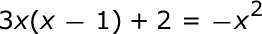#### Question 5 5. Solve the following inequality.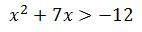### Page 2

#### Question 6 6. Find the roots of the equation below.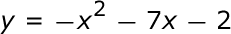#### Question 7 7. Find the roots of the equation.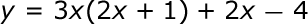### Page 3

#### Question 12 12. What are the zeros in the following quadratic equation?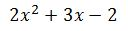#### Question 13 13. Which statement is true about the inequality below?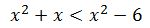### Page 4

#### Question 17 17. What is the b-value of the quadratic equation below?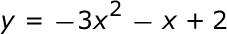#### Question 18 18. Solve this inequality.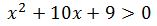#### Question 19 19. Use the quadratic formula to find the solutions to the equation below.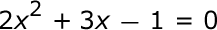#### Question 20 20. Which of the following are the solutions to the equation below?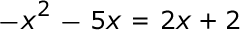### Page 5

#### Question 25 25. Solve the following equation.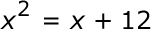#### Algebra II: Quadratic Equations Chapter Exam Instructions

Choose your answers to the questions and click 'Next' to see the next set of questions. You can skip questions if you would like and come back to them later with the yellow "Go To First Skipped Question" button. When you have completed the practice exam, a green submit button will appear. Click it to see your results. Good luck!

Support